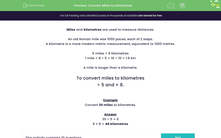# Convert Miles to Kilometres

In this worksheet, students must convert distances from miles to kilometres.Key stage:  KS 2

Curriculum topic:   Measurement

Curriculum subtopic:   Convert Miles/Kilometres

Difficulty level:#### Worksheet Overview

Miles and kilometres are used to measure distances.

An old Roman mile was 1000 paces, each of 2 steps.

A kilometre is a more modern metric measurement, equivalent to 1000 metres.

5 miles = 8 kilometres

1 mile = 8 ÷ 5 = 16 ÷ 10 = 1.6 km

A mile is longer than a kilometre.

To convert miles to kilometres

÷ 5 and × 8.

Example

Convert 30 miles to kilometres.